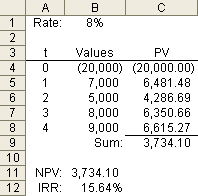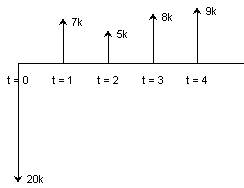Companies use the Net Present Value (NPV) calculation to help decide whether an investment will add value in the long run, to compare different investment options, and to decide whether to introduce a new product. In addition to explaining how to calculate NPV and IRR, you can download a Free Excel NPV Calculator to help you see how to set up your own financial analysis spreadsheet.

Unlike a break-even analysis which is based on a Net Income of 0, the NPV includes a discount rate, or the rate or return that could be earned on an alternative investment in the financial market for example. If the NPV calculation is > 0, then the new product line (or whatever it is you are analyzing) may be worth pursuing.

The Internal Rate of Return or IRR is another useful metric for analyzing an investment. The IRR function in Excel uses the same series of cash flows as the NPV, but it must have at least one negative (usually at t=0) and one positive value and it requires an initial guess at the rate because the calculation is iterative. The NPV Calculator spreadsheet includes the IRR calculation as well.

## NPV Calculator with IRR, XNPV, and XIRR

for Excel, Google Sheets and OpenOffice

⤓ Excel (.xlsx)
For: Excel 2007 or later

### Other Versions

License: Private Use (not for distribution or resale)

"No installation, no macros - just a simple spreadsheet" - by

### Description

The workbook contains two worksheets:

1) The NPV Calculator worksheet shown in the screenshot above lets you calculate NPV and IRR for multiple series of cash flows.

2) The XIRR, XNPV Calculator in the screenshot on the right uses Excel's XNPV and XIRR functions to calculate Net Present Value and Internal Rate of Return for a non-periodic series of cash flows (based on the dates).

- See how to set up the NPV and IRR cash flows and formulas.

## How to Calculate IRR and NPV in Excel

To calculate NPV or IRR, you first need to have a predicted or estimated series of periodic cash flows. This will usually involve some initial lump payment as the initial investment (negative cash flow) at time t=0, followed by both inflows (income) and outflows (payments) at regular intervals t=1, t=2, t=3, etc. The image below shows an example.Fig 1. Sample series of cash flows
with NPV and IRR calculation.Fig 2. Cash flow diagram.

Net Present Value is defined as the "difference between the present value (PV) of the future cash flows from an investment and the amount of investment"  (emphasis added).

This is an excellent definition because it explains why the NPV formula in Excel is not really complete, and what you need to do to use it correctly. The word "Net" in NPV implies subtracting something from something else. The Excel NPV function really only calculates the sum of the present value of future cash flows. Note the emphasis on "future", which means cash flows starting at time t=1 or 1 period in the future from the present time t=0.

To calculate NPV, you must also subtract the value of the initial investment, or in other words, add the cash flow at time t=0 (which is a negative value). So in Figure 1 above, the equation for NPV is:

```=NPV(rate, values_t1_to_tn) + value_t0
=NPV(B1,B5:B8)+B4```

The formula for the internal rate of return (IRR) in Figure 1 is:

```=IRR(values_t0_to_tn, guess)
=IRR(B4:B8,0.1)```

It would be a good idea to read the Help document on the NPV and IRR functions, so that you can understand some of the limitations and requirements.

It is educational to note that the NPV formula is identical to calculating the present value of all future cash flows using the PV formula and summing them as shown in Figure 1. This can also be done using an array formula as explained by Timothy Mayers in reference  below. The array formula is actually more powerful than the NPV function, because you can include the initial cash flow at t=0 to avoid having to add it in separately, and you can have multiple values for the same period or even have the periods out of order.

`=SUM(PV(rate, periods_t0_to_tn, 0, -values))`

## Using the XNPV and XIRR Formulas to Calculate NPV and IRR

The second worksheet in the NPV Calculator spreadsheet is set up to help you calculate the Net Present Value and Internal Rate of Return for a series of scheduled cash flows that are non-periodic. The XNPV and XIRR functions require you to enter dates in addition to the cash flow values and the discount rate. They use a 365-day year to calculate present value based on an initial negative value (investment).

XIRR is to XNPV as IRR is to NPV

The following array formula can be used in place of the XNPV formula if you need to use a 360-day year or don't want to require the use of the Analysis ToolPak. Because it is an array formula, you need to press Ctrl+Shift+Enter after adding or editing it.

`=SUM(values/((1+rate)^((dates-INDEX(dates,1))/365)))`

### References

•  Definition of Net Present Value at http://www.businessdictionary.com/definition/net-present-value-NPV.html
•  The NPV Function Doesn't Calculate Net Present Value by Timothy R. Mayes at http://www.tvmcalcs.com/index.php/blog/comments/the_npv_function_doesnt_calculate_net_present_value/ - Explains how to correctly use the NPV function.
• Time Value Function Tutorial by Timothy R. Mayes at http://www.tvmcalcs.com/calculators/excel_tvm_functions/excel_tvm_functions_page3